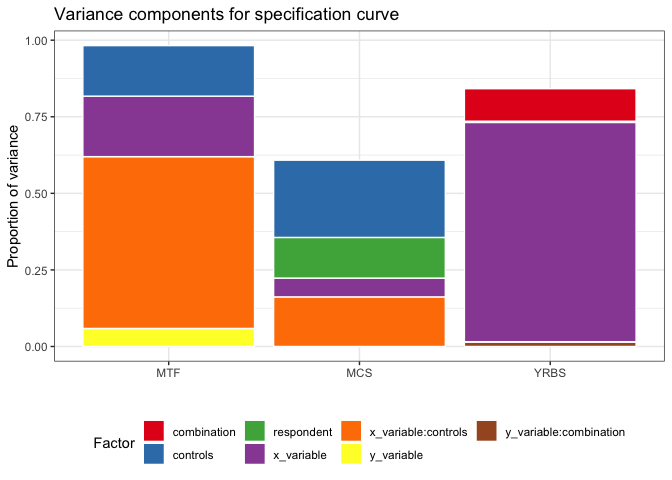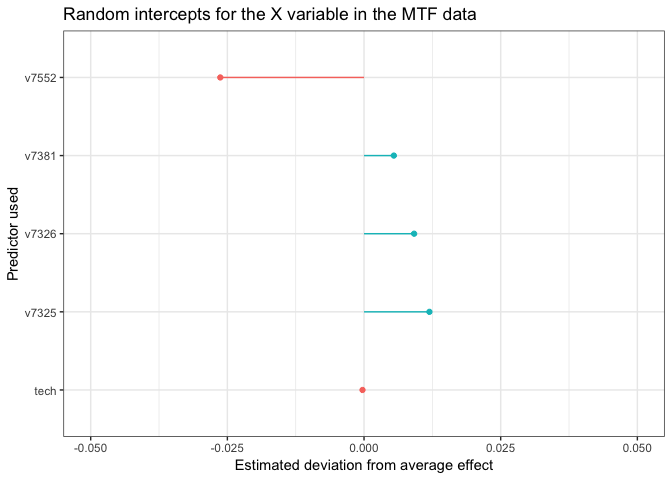# Getting more information out of the specification curve

Michael Scharkow | 2019-01-15

TL;DR: You can extract various interesting bits of information from a specification curve analysis (SCA) if you analyze it as a factorial experiment using multilevel regression.

## Understanding the specification curve

Yesterday, Amy Orben and Andy Przybylski published a very, very nice paper on the association between adolescent well-being and digital technology use (open access version). While the topic and results are interesting and relevant in their own rights, I want to look at their analytical approach - the specification curve, originally developed by Simonsohn, Simmons and Nelson (2015). The basic idea is that, instead of selectively making analytical decisions such as adding or removing covariates, subsetting the data or using different outcomes, we can try all forking paths and see where that leads us. In short, a specification curve analysis is a factorial experiment which systematically varies analytical decisions and saves the quantity of interest, e.g. a regression coefficent or p-value. The results typically look like this

Specification curve by Orben & Przybylski, 2019

There’s a lot going on the this graph, and I find myself staring at the bottom half a lot longer than I’d like. The original eyeballing strategy advocated by Simonsohn et al. is to look at the proportion of significant results as a sort of robustness check. Or one could, as Andy does in this tweet point out that an effect larger or smaller than X is only found in some small percentage of all analyses, which makes these findings a little suspicious. Or one could look at the average or median effect as a kind-of-fake meta-analytic result. All of this focuses exclusively on the outcome, i.e. the statistical significance of the finding or the effect size.

## A different perspective on the data

Another way to look at the graph above is to focus on the impact of different strategies or decisions, and where they tend to cluster results-wise. The bottom half of the figure demonstrates, for example, that strong negative effects occur only if one ignores important covariates. However, eyeballing these plots gets more difficult if we include many more analytical decisions or if these decisions have many different variants.

I want to suggest another way of summarising the results of the specification curve: variance components. Remember that essentially we are analyzing a large factorial experiment, and that a well-known strategy to summarise the relative importance of batches of variables is to look at the share of variance in the outcome (the regression coefficient) that different factors account for. This makes the specification curve more informative, since we can quantify in a straightforward way where the variation in results comes from. Consequently, we know were to direct our attention in terms of validation and standardization (and also where it is most promising to p-hack!)

## Motivating example

Let’s look at the MTF dataset, one of three used in Orben & Przybylski, first. Since Amy and Andy are good scientists who care about reproducibility, the data and code from the specification curve analysis can be found on the paper’s OSF project page. For now, I only use the result data sets.

library(lme4)
library(sjstats)
library(tidyverse)
theme_set(theme_bw())

load("2_2_sca_mtf_results_subset1.rda")
load("2_3_sca_mcs_results.rda")
load("2_1_sca_yrbs_results.rda")

names(results_mtf_ds_1)
##  "x_variable"     "y_variable"     "controls"       "effect"
##  "t_value"        "p_value"        "standard_error" "number"
##  "rsqrd"

In case of the MTF data set, the authors varied the operationalisation of the $$X$$ and $$Y$$ variables as well as the inclusion of control variables. Borrowing a strategy from Andrew Gelman, we can simply include all factors of the specification curve, i.e. all analytical choices, as random effects in an otherwise empty multilevel model, with the effect as the outcome variable. I chose the standardized regression coefficient (effect) as the quantity of interest, but you could just as well look at the t- or p-values (don’t!).

mtf_model_1 = results_mtf_ds_1 %>%
mutate(x_variable =  map_chr(x_variable, paste, collapse=","),
y_variable =  map_chr(y_variable, paste, collapse=",")) %>%
lmer(effect ~ 1 + (1|x_variable)+(1|y_variable) + (1|controls), .)
summary(mtf_model_1)
## Linear mixed model fit by REML ['lmerMod']
## Formula: effect ~ 1 + (1 | x_variable) + (1 | y_variable) + (1 | controls)
##    Data: .
##
## REML criterion at convergence: -246235.3
##
## Scaled residuals:
##     Min      1Q  Median      3Q     Max
## -2.8431 -0.7372  0.0780  0.7504  3.2746
##
## Random effects:
##  Groups     Name        Variance  Std.Dev.
##  y_variable (Intercept) 1.688e-05 0.004109
##  x_variable (Intercept) 2.372e-04 0.015400
##  controls   (Intercept) 1.377e-04 0.011733
##  Residual               1.317e-04 0.011476
## Number of obs: 40950, groups:
## y_variable, 4095; x_variable, 5; controls, 2
##
## Fixed effects:
##             Estimate Std. Error t value
## (Intercept) -0.00730    0.01078  -0.677

We see that there are many variations in the $$Y$$ variable (well-being), but relatively few variants of the technology use measure ($$X$$), and only a binary controls yes/no variable. We can also see that the estimated mean effect is practically zero. More interesting are the random effect variances, which can be transformed into an easily interpretable intra-class correlation coefficent.

icc(mtf_model_1)
##
## Intraclass Correlation Coefficient for Linear mixed model
##
## Family : gaussian (identity)
## Formula: effect ~ 1 + (1 | x_variable) + (1 | y_variable) + (1 | controls)
##
##   ICC (y_variable): 0.0323
##   ICC (x_variable): 0.4531
##     ICC (controls): 0.2630

While there are so many different ways to compute the well-being score, the choice of $$Y$$ accounts for only three percent of the variance in the regression coefficient. Conversely, the choice of media use measure accounts for almost half of the variance in effects, and the control variable for another quarter of the variance. The remaining variance can be understood as either residuals or simply unmodeled interactions between the factors. Let’s see what happens if we add an interaction of $$X$$ and the controls variable.

mtf_model_2 = results_mtf_ds_1 %>%
mutate(x_variable =  map_chr(x_variable, paste, collapse=","),
y_variable =  map_chr(y_variable, paste, collapse=",")) %>%
lmer(effect ~ 1 + (1|x_variable)+(1|y_variable) + (1|controls) + (1|x_variable:controls), .)
icc(mtf_model_2)
##
## Intraclass Correlation Coefficient for Linear mixed model
##
## Family : gaussian (identity)
## Formula: effect ~ 1 + (1 | x_variable) + (1 | y_variable) + (1 | controls) + (1 | x_variable:controls)
##
##            ICC (y_variable): 0.0589
##   ICC (x_variable:controls): 0.5609
##            ICC (x_variable): 0.1975
##              ICC (controls): 0.1650

The results change, and unsurprisingly, we see that playing around with both $$X$$ and the control variable (so basically all predictors in the original regression model) has a very large influence on the effects found.

We can quickly estimate similar null models for the YRBS and MCS data, which both include additional forking paths in terms of model specification.

yrbs_model = results_yrbs_sca %>%
mutate(x_variable =  map_chr(x_variable, paste, collapse=","),
y_variable =  map_chr(y_variable, paste, collapse=",")) %>%
lmer(effect ~ 1 + (1|x_variable)+(1|y_variable) + (1|combination) +
(1|y_variable:combination) + (1|controls), .)

icc(yrbs_model)
##
## Intraclass Correlation Coefficient for Linear mixed model
##
## Family : gaussian (identity)
## Formula: effect ~ 1 + (1 | x_variable) + (1 | y_variable) + (1 | combination) + (1 | y_variable:combination) + (1 | controls)
##
##   ICC (y_variable:combination): 0.0146
##               ICC (y_variable): 0.0011
##               ICC (x_variable): 0.7158
##                 ICC (controls): 0.0039
##              ICC (combination): 0.1062
mcs_model = results_mcs_sca_total %>%
mutate(x_variable =  map_chr(x_variable, paste, collapse=","),
y_variable =  map_chr(y_variable, paste, collapse=",")) %>%
lmer(effect ~ 1 + (1|x_variable)+(1|y_variable) + (1|respondent) +
(1|x_variable:controls) + (1|controls), .)
## singular fit
icc(mcs_model)
##
## Intraclass Correlation Coefficient for Linear mixed model
##
## Family : gaussian (identity)
## Formula: effect ~ 1 + (1 | x_variable) + (1 | y_variable) + (1 | respondent) + (1 | x_variable:controls) + (1 | controls)
##
##            ICC (y_variable): 0.0000
##   ICC (x_variable:controls): 0.1619
##            ICC (x_variable): 0.0614
##              ICC (controls): 0.2522
##            ICC (respondent): 0.1328

In the YRBS data, the choice of $$X$$ seems especially consequential, while the MCS data show that asking respondents vs. others about the respondents’ well-being can influence the findings a lot. In all cases, it seems that the choice of well-being measure is relatively inconsequential. Note that I spent about 3 minutes for the specification of the random effects and might have omitted some really important interactions.

Let’s summarise our findings graphically using a quick and dirty 3-D pie chart~ colorful bar plot.

list(mtf_model_2, mcs_model, yrbs_model) %>% map(icc) %>%
map(data.frame) %>% map_df(rownames_to_column, "Factor", .id =  "data") %>%
mutate(data = factor(data, labels = c("MTF", "MCS", "YRBS"))) %>%
rename(prop_var = .x..i..) %>%
ggplot(aes(x = data, y = prop_var, fill = Factor)) +
geom_bar(stat="identity",  color="white")+scale_fill_brewer(palette="Set1")+
theme(legend.position = "bottom")+ylab("Proportion of variance")+ xlab("")+
ggtitle("Variance components for specification curve")## Bonus: Inspecting large individual effects

If you are still interested in the effects of specific decisions, e.g. which $$X$$ variable leads to the largest (smallest) effects, you can easily extract the information from the lmer model. The code below selects the 10 variants with the largest absolute deviation from the estimated average effect. In this case, there are only 5 measures of digital media use available, but as an experienced scholar in the field of negative media effects, I’m totally sure that v7552 is the most valid and reliable measure, whatever v7552 is.

ranef(mtf_model_1)\$x_variable %>% as_tibble(rownames = "x_variable") %>%
rename(effect = (Intercept)) %>% arrange(-abs(effect)) %>% slice(1:10) %>%
ggplot(aes(x = x_variable, y = effect, color = effect > 0)) + geom_point() +
geom_segment(aes(yend = 0, xend = x_variable))+coord_flip(ylim = c(-.05, .05))+
theme(legend.position = "none")+
labs(x = "Predictor used", y = "Estimated deviation from average effect",
title = "Random intercepts for the X variable in the MTF data")And that’s that. Thanks to Amy Orben and Andrew Przybylski for making the data available, and for an inspiring paper.